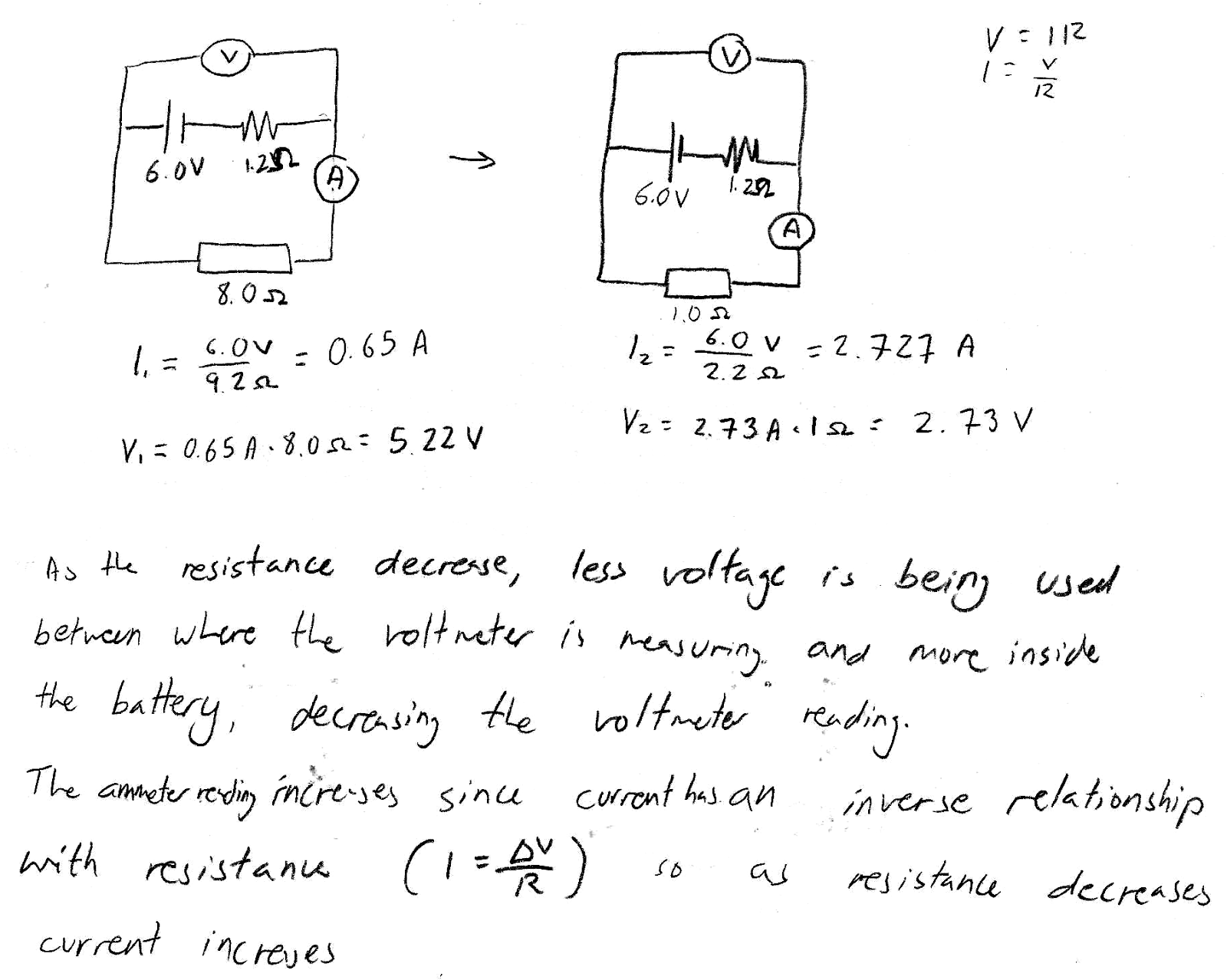## 20170507

### Physics midterm problem: pencil lead variable resistor

Physics 205B Midterm 2, spring semester 2017
Cuesta College, San Luis Obispo, CAA real battery with an emf of 6.0 V and an internal resistance of r = 1.2 Ω is attached to an ideal voltmeter, and is connected to an ideal ammeter and a pencil lead that acts as a variable resistor. If the amount of pencil lead between the contacts is shortened such that its resistance is reduced from 8.0 Ω to 1.0 Ω, discuss why the voltmeter reading will decrease while the ammeter reading will increase. Show your work and explain your reasoning using Kirchhoff's rules, Ohm's law, and properties of ammeters and voltmeters.

• p:
Correct. Explains why the voltmeter reading will decrease while the ammeter reading will increase as the amount of pencil lead between the contacts is shortened by discussing:
1. the decrease in the resistance of the pencil lead resistor will reduce the equivalent resistance of the circuit (pencil lead and internal resistance are in series), such that from applying Ohm's law the amount of current passing everywhere through the circuit will increase, resulting in a higher ammeter reading; and
2. the voltmeter measures the potential difference of the 6.0 V rise from the emf and the voltage drop Ir from the internal resistance, such that an increase in current will result in a lower voltage reading ΔV = +ε − Ir.
• (May instead discuss how the voltmeter is equivalently measuring the voltage drop ΔV = −IR across the pencil lead resistor, but must clearly show that the eight-fold decrease in the resistance (from 8.0 Ω to 1.0 Ω) will be larger than the corresponding approximate four-fold increase in current (0.65 A to 2.7 A) to result in a lower voltage reading.)
• r:
Nearly correct, but includes minor math errors.
• t:
Nearly correct, but approach has conceptual errors, and/or major/compounded math errors. At least numerically or qualitatively demonstrates how current would increase, but does not definitely show why voltmeter reading would decrease.
• v:
Implementation of right ideas, but in an inconsistent, incomplete, or unorganized manner. Some attempt at applying Kirchhoff's rules, Ohm's law, and equivalent resistance.
• x:
Implementation of ideas, but credit given for effort rather than merit. Approach other than that of applying Kirchhoff's rules, Ohm's law, and properties of ammeters and voltmeters.
• y:
Irrelevant discussion/effectively blank.
• z:
Blank.
Sections 30882, 30883
Exam code: midterm02GruT
p: 12 students
r: 0 students
t: 8 students
v: 8 students
x: 1 student
y: 0 students
z: 0 students

A sample "p" response (from student 1412):Another sample "p" response (from student 2643):A sample "x" response (from student 9319):